# ISEE Upper Level Math : How to find the perimeter of a rhombus

## Example Questions

### Example Question #1 : How To Find The Perimeter Of A Rhombus

One side of a rhombus measures 2 yards. Which of the following is equal to the perimeter?

All of the other choices give the correct perimeter.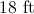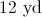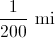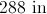Explanation:

A rhombus has four sides of equal measure, so the perimeter of a rhombus with sidelength 2 yards is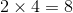yards.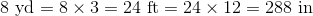Also,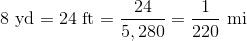The only choice that agrees with any of these measures is 288 inches, the correct choice.

### Example Question #7 : Rhombuses

One side of a rhombus measures. Give its perimeter in terms of.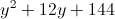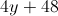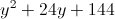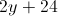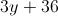A rhombus has four sides of equal measure, so the perimeter of a rhombus with sidelength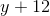is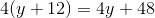.